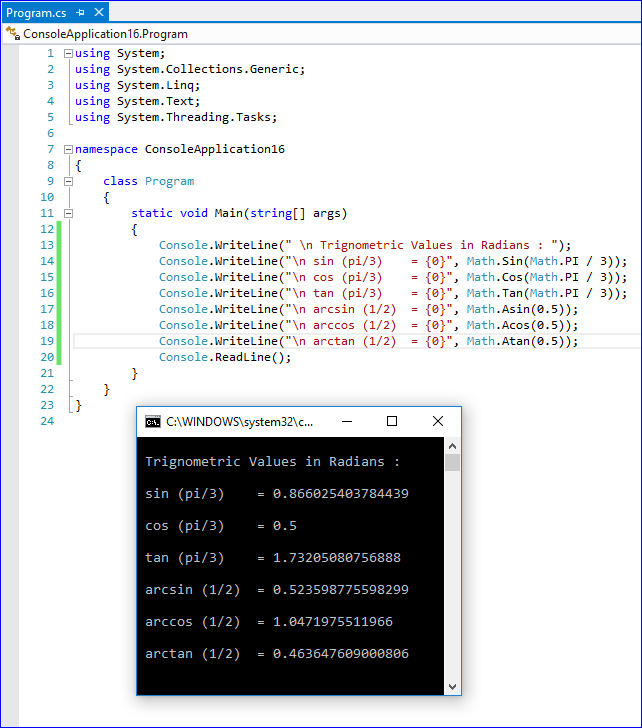#### Write a Program to Illustrate Trigonometry Angles in Radians in C# ?

Total Post:46

Points:324

506  View(s)
Ratings:
Rate this:

Like:-

Sin, cos, Tan, Cot, Sec, Cosec. etc

1.##### Re: Write a Program to Illustrate Trigonometry Angles in Radians in C# ?

```using System;
using System.Collections.Generic;
using System.Linq;
using System.Text;

namespace ConsoleApplication17
{
class Program
{
static void Main(string[] args)
{
Console.WriteLine(" \n Trignometric Values in Radians : ");
Console.WriteLine("\n sin (pi/3)    = {0}", Math.Sin(Math.PI / 3));
Console.WriteLine("\n cos (pi/3)    = {0}", Math.Cos(Math.PI / 3));
Console.WriteLine("\n tan (pi/3)    = {0}", Math.Tan(Math.PI / 3));
Console.WriteLine("\n arcsin (1/2)  = {0}", Math.Asin(0.5));
Console.WriteLine("\n arccos (1/2)  = {0}", Math.Acos(0.5));
Console.WriteLine("\n arctan (1/2)  = {0}", Math.Atan(0.5));